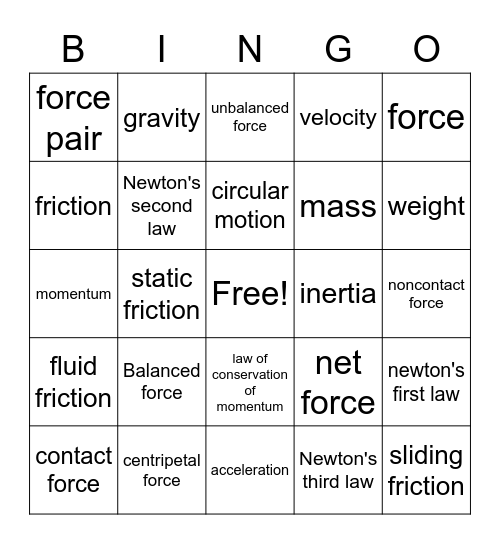Newton's laws of motionThis bingo card has a free space and 24 words: Balanced force, centripetal force, circular motion, contact force, force, force pair, friction, gravity, inertia, law of conservation of momentum, mass, momentum, net force, newton's first law, Newton's second law, Newton's third law, noncontact force, unbalanced force, weight, static friction, sliding friction, fluid friction, velocity and acceleration.

Play Online